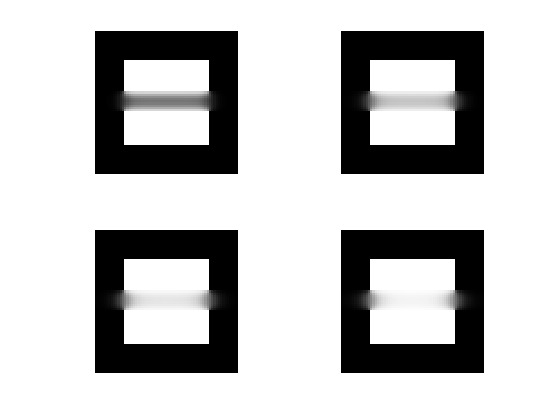$\newcommand{\NN}{\mathbb{N}} \newcommand{\CC}{\mathbb{C}} \newcommand{\GG}{\mathbb{G}} \newcommand{\LL}{\mathbb{L}} \newcommand{\PP}{\mathbb{P}} \newcommand{\QQ}{\mathbb{Q}} \newcommand{\RR}{\mathbb{R}} \newcommand{\VV}{\mathbb{V}} \newcommand{\ZZ}{\mathbb{Z}} \newcommand{\FF}{\mathbb{F}} \newcommand{\KK}{\mathbb{K}} \newcommand{\UU}{\mathbb{U}} \newcommand{\EE}{\mathbb{E}} \newcommand{\Aa}{\mathcal{A}} \newcommand{\Bb}{\mathcal{B}} \newcommand{\Cc}{\mathcal{C}} \newcommand{\Dd}{\mathcal{D}} \newcommand{\Ee}{\mathcal{E}} \newcommand{\Ff}{\mathcal{F}} \newcommand{\Gg}{\mathcal{G}} \newcommand{\Hh}{\mathcal{H}} \newcommand{\Ii}{\mathcal{I}} \newcommand{\Jj}{\mathcal{J}} \newcommand{\Kk}{\mathcal{K}} \newcommand{\Ll}{\mathcal{L}} \newcommand{\Mm}{\mathcal{M}} \newcommand{\Nn}{\mathcal{N}} \newcommand{\Oo}{\mathcal{O}} \newcommand{\Pp}{\mathcal{P}} \newcommand{\Qq}{\mathcal{Q}} \newcommand{\Rr}{\mathcal{R}} \newcommand{\Ss}{\mathcal{S}} \newcommand{\Tt}{\mathcal{T}} \newcommand{\Uu}{\mathcal{U}} \newcommand{\Vv}{\mathcal{V}} \newcommand{\Ww}{\mathcal{W}} \newcommand{\Xx}{\mathcal{X}} \newcommand{\Yy}{\mathcal{Y}} \newcommand{\Zz}{\mathcal{Z}} \newcommand{\al}{\alpha} \newcommand{\la}{\lambda} \newcommand{\ga}{\gamma} \newcommand{\Ga}{\Gamma} \newcommand{\La}{\Lambda} \newcommand{\Si}{\Sigma} \newcommand{\si}{\sigma} \newcommand{\be}{\beta} \newcommand{\de}{\delta} \newcommand{\De}{\Delta} \renewcommand{\phi}{\varphi} \renewcommand{\th}{\theta} \newcommand{\om}{\omega} \newcommand{\Om}{\Omega} \renewcommand{\epsilon}{\varepsilon} \newcommand{\Calpha}{\mathrm{C}^\al} \newcommand{\Cbeta}{\mathrm{C}^\be} \newcommand{\Cal}{\text{C}^\al} \newcommand{\Cdeux}{\text{C}^{2}} \newcommand{\Cun}{\text{C}^{1}} \newcommand{\Calt}{\text{C}^{#1}} \newcommand{\lun}{\ell^1} \newcommand{\ldeux}{\ell^2} \newcommand{\linf}{\ell^\infty} \newcommand{\ldeuxj}{{\ldeux_j}} \newcommand{\Lun}{\text{\upshape L}^1} \newcommand{\Ldeux}{\text{\upshape L}^2} \newcommand{\Lp}{\text{\upshape L}^p} \newcommand{\Lq}{\text{\upshape L}^q} \newcommand{\Linf}{\text{\upshape L}^\infty} \newcommand{\lzero}{\ell^0} \newcommand{\lp}{\ell^p} \renewcommand{\d}{\ins{d}} \newcommand{\Grad}{\text{Grad}} \newcommand{\grad}{\text{grad}} \renewcommand{\div}{\text{div}} \newcommand{\diag}{\text{diag}} \newcommand{\pd}{ \frac{ \partial #1}{\partial #2} } \newcommand{\pdd}{ \frac{ \partial^2 #1}{\partial #2^2} } \newcommand{\dotp}{\langle #1,\,#2\rangle} \newcommand{\norm}{|\!| #1 |\!|} \newcommand{\normi}{\norm{#1}_{\infty}} \newcommand{\normu}{\norm{#1}_{1}} \newcommand{\normz}{\norm{#1}_{0}} \newcommand{\abs}{\vert #1 \vert} \newcommand{\argmin}{\text{argmin}} \newcommand{\argmax}{\text{argmax}} \newcommand{\uargmin}{\underset{#1}{\argmin}\;} \newcommand{\uargmax}{\underset{#1}{\argmax}\;} \newcommand{\umin}{\underset{#1}{\min}\;} \newcommand{\umax}{\underset{#1}{\max}\;} \newcommand{\pa}{\left( #1 \right)} \newcommand{\choice}{ \left\{ \begin{array}{l} #1 \end{array} \right. } \newcommand{\enscond}{ \left\{ #1 \;:\; #2 \right\} } \newcommand{\qandq}{ \quad \text{and} \quad } \newcommand{\qqandqq}{ \qquad \text{and} \qquad } \newcommand{\qifq}{ \quad \text{if} \quad } \newcommand{\qqifqq}{ \qquad \text{if} \qquad } \newcommand{\qwhereq}{ \quad \text{where} \quad } \newcommand{\qqwhereqq}{ \qquad \text{where} \qquad } \newcommand{\qwithq}{ \quad \text{with} \quad } \newcommand{\qqwithqq}{ \qquad \text{with} \qquad } \newcommand{\qforq}{ \quad \text{for} \quad } \newcommand{\qqforqq}{ \qquad \text{for} \qquad } \newcommand{\qqsinceqq}{ \qquad \text{since} \qquad } \newcommand{\qsinceq}{ \quad \text{since} \quad } \newcommand{\qarrq}{\quad\Longrightarrow\quad} \newcommand{\qqarrqq}{\quad\Longrightarrow\quad} \newcommand{\qiffq}{\quad\Longleftrightarrow\quad} \newcommand{\qqiffqq}{\qquad\Longleftrightarrow\qquad} \newcommand{\qsubjq}{ \quad \text{subject to} \quad } \newcommand{\qqsubjqq}{ \qquad \text{subject to} \qquad }$

This tour explores the use of gradient descent method for unconstrained and constrained optimization of a smooth function

## Installing toolboxes and setting up the path.

You need to download the following files: signal toolbox and general toolbox.

You need to unzip these toolboxes in your working directory, so that you have toolbox_signal and toolbox_general in your directory.

For Scilab user: you must replace the Matlab comment '%' by its Scilab counterpart '//'.

Recommandation: You should create a text file named for instance numericaltour.sce (in Scilab) or numericaltour.m (in Matlab) to write all the Scilab/Matlab command you want to execute. Then, simply run exec('numericaltour.sce'); (in Scilab) or numericaltour; (in Matlab) to run the commands.

Execute this line only if you are using Matlab.

getd = @(p)path(p,path); % scilab users must *not* execute this


Then you can add the toolboxes to the path.

getd('toolbox_signal/');
getd('toolbox_general/');


## Gradient Descent for Unconstrained Problems

We consider the problem of finding a minimum of a function $$f$$, hence solving $\umin{x \in \RR^d} f(x)$ where $$f : \RR^d \rightarrow \RR$$ is a smooth function.

Note that the minimum is not necessarily unique. In the general case, $$f$$ might exhibit local minima, in which case the proposed algorithms is not expected to find a global minimizer of the problem. In this tour, we restrict our attention to convex function, so that the methods will converge to a global minimizer.

The simplest method is the gradient descent, that computes $x^{(k+1)} = x^{(k)} - \tau_k \nabla f(x^{(k)}),$ where $$\tau_k>0$$ is a step size, and $$\nabla f(x) \in \RR^d$$ is the gradient of $$f$$ at the point $$x$$, and $$x^{(0)} \in \RR^d$$ is any initial point.

In the convex case, if $$f$$ is of class $$C^2$$, in order to ensure convergence, the step size should satisfy $0 < \tau_k < \frac{2}{ \sup_x \norm{Hf(x)} }$ where $$Hf(x) \in \RR^{d \times d}$$ is the Hessian of $$f$$ at $$x$$ and $$\norm{\cdot}$$ is the spectral operator norm (largest eigenvalue).

We consider a simple problem, corresponding to the minimization of a 2-D quadratic form $f(x) = \frac{1}{2} \pa{ x_1^2 + \eta x_2^2, }$ where $$\eta>0$$ controls the anisotropy, and hence the difficulty, of the problem.

Anisotropy parameter $$\eta$$.

eta = 10;


Function $$f$$.

f = @(x)( x(1)^2 + eta*x(2)^2 ) /2;


Background image of the function.

t = linspace(-.7,.7,101);
[u,v] = meshgrid(t,t);
F = ( u.^2 + eta*v.^2 )/2 ;


Display the function as a 2-D image.

clf; hold on;
imagesc(t,t,F); colormap jet(256);
contour(t,t,F, 20, 'k');
axis off; axis equal;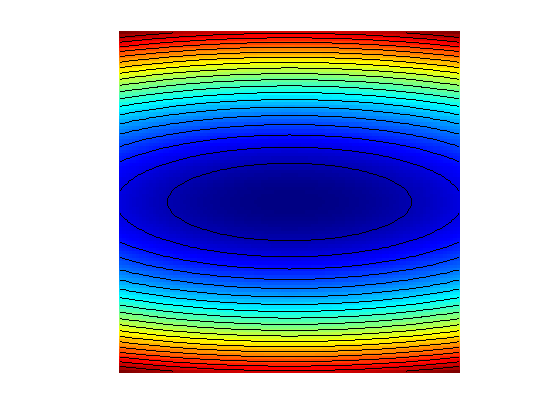Gradf = @(x)[x(1); eta*x(2)];


The step size should satisfy $$\tau_k < 2/\eta$$. We use here a constrant step size.

tau = 1.8/eta;


Exercice 1: (check the solution) Perform the gradient descent using a fixed step size $$\tau_k=\tau$$. Display the decay of the energy $$f(x^{(k)})$$ through the iteration. Save the iterates so that X(:,k) corresponds to $$x^{(k)}$$.

exo1;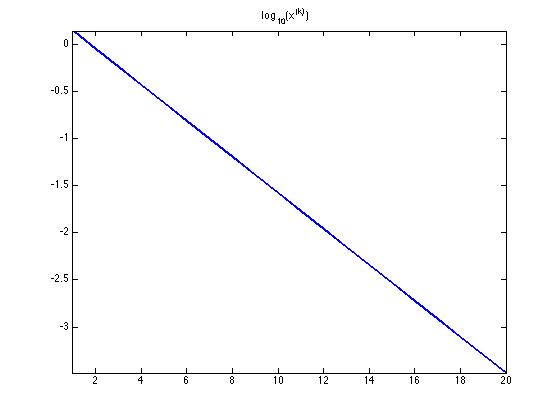Display the iterations.

clf; hold on;
imagesc(t,t,F); colormap jet(256);
contour(t,t,F, 20, 'k');
h = plot(X(1,:), X(2,:), 'k.-');
set(h, 'LineWidth', 2);
set(h, 'MarkerSize', 15);
axis off; axis equal;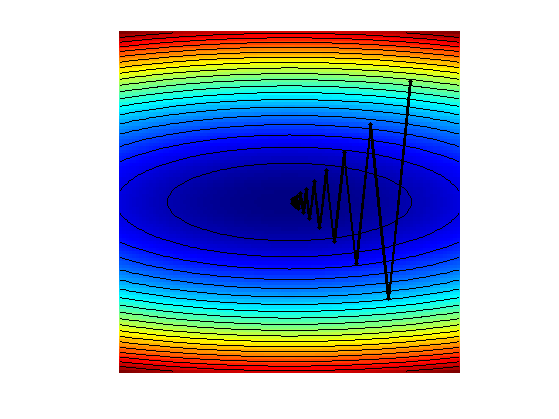Exercice 2: (check the solution) Display the iteration for several different step sizes.

exo2;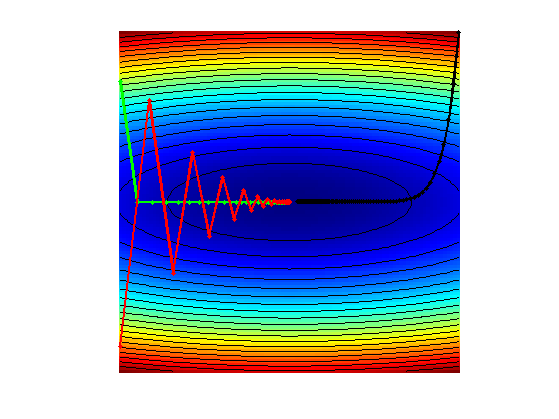## Gradient and Divergence of Images

Local differential operators like gradient, divergence and laplacian are the building blocks for variational image processing.

Load an image $$x_0 \in \RR^N$$ of $$N=n \times n$$ pixels.

n = 256;


Display it.

clf;
imageplot(x0);For a continuous function $$g$$, the gradient reads $\nabla g(s) = \pa{ \pd{g(s)}{s_1}, \pd{g(s)}{s_2} } \in \RR^2.$ (note that here, the variable $$s$$ denotes the 2-D spacial position).

We discretize this differential operator on a discrete image $$x \in \RR^N$$ using first order finite differences. $(\nabla x)_i = ( x_{i_1,i_2}-x_{i_1-1,i_2}, x_{i_1,i_2}-x_{i_1,i_2-1} ) \in \RR^2.$ Note that for simplity we use periodic boundary conditions.

Compute its gradient, using finite differences.

grad = @(x)cat(3, x-x([end 1:end-1],:), x-x(:,[end 1:end-1]));


One thus has $$\nabla : \RR^N \mapsto \RR^{N \times 2}.$$

v = grad(x0);


One can display each of its components.

clf;
imageplot(v(:,:,1), 'd/dx', 1,2,1);
imageplot(v(:,:,2), 'd/dy', 1,2,2);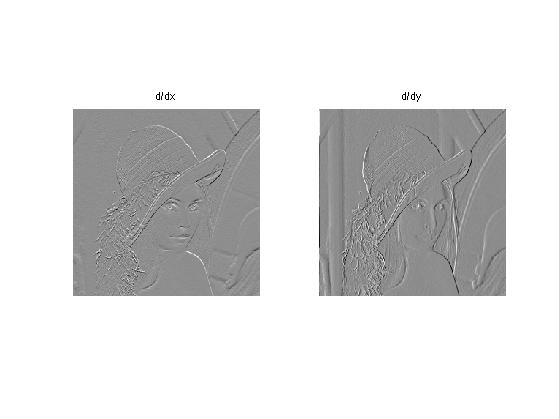One can also display it using a color image.

clf;
imageplot(v);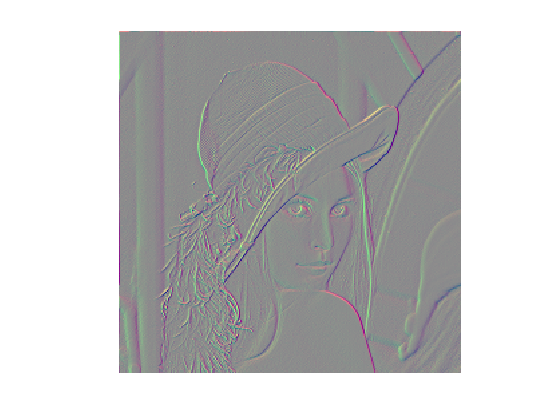One can display its magnitude $$\norm{(\nabla x)_i}$$, which is large near edges.

clf;
imageplot( sqrt( sum3(v.^2,3) ) );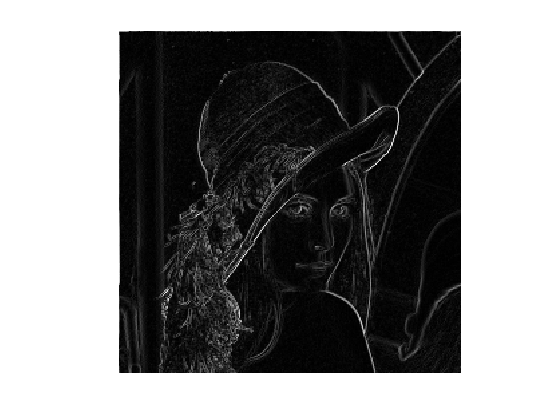The divergence operator maps vector field to images. For continuous vector fields $$v(s) \in \RR^2$$, it is defined as $\text{div}(v)(s) = \pd{v_1(s)}{s_1} + \pd{v_2(s)}{s_2} \in \RR.$ (note that here, the variable $$s$$ denotes the 2-D spacial position). It is minus the adjoint of the gadient, i.e. $$\text{div} = - \nabla^*$$.

It is discretized, for $$v=(v^1,v^2)$$ as $\text{div}(v)_i = v^1_{i_1+1,i_2} - v^1_{i_1,i_2} + v^2_{i_1,i_2+1} - v^2_{i_1,i_2} .$

div = @(v)v([2:end 1],:,1)-v(:,:,1) + v(:,[2:end 1],2)-v(:,:,2);


The Laplacian operatore is defined as $$\Delta=\text{div} \circ \nabla = -\nabla^* \circ \nabla$$. It is thus a negative symmetric operator.

delta = @(x)div(grad(x));


Display $$\Delta x_0$$.

clf;
imageplot(delta(x0));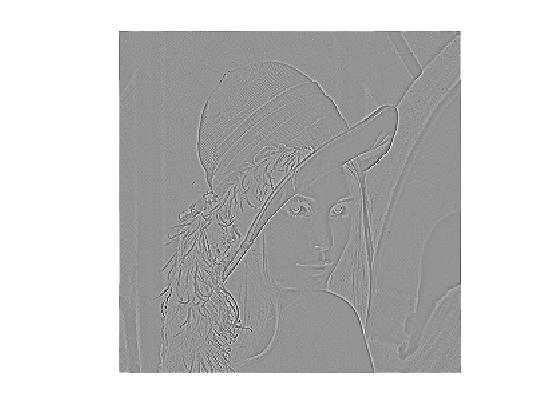Check that the relation $$\norm{\nabla x} = - \dotp{\Delta x}{x}.$$

dotp = @(a,b)sum(a(:).*b(:));

Should be 0: 000


## Gradient Descent in Image Processing

We consider now the problem of denoising an image $$y \in \RR^d$$ where $$d = n \times n$$ is the number of pixels ($$n$$ being the number of rows/columns in the image).

Add noise to the original image, to simulate a noisy image.

sigma = .1;
y = x0 + randn(n)*sigma;


Display the noisy image $$y$$.

clf;
imageplot(clamp(y));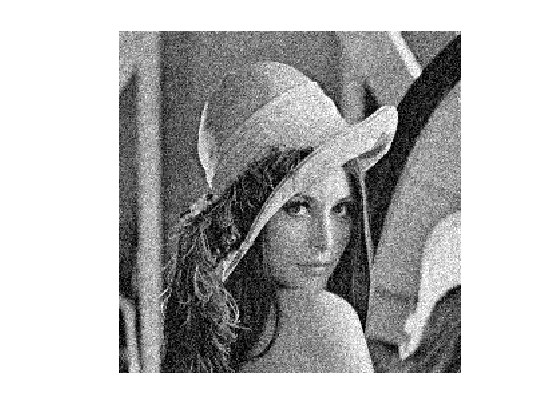Denoising is obtained by minimizing the following functional $\umin{x \in \RR^d} f(x) = \frac{1}{2} \norm{y-x}^2 + \la J_\epsilon(x)$ where $$J_\epsilon(x)$$ is a smoothed total variation of the image. $J_\epsilon(x) = \sum_i \norm{ (G x)_i }_{\epsilon}$ where $$(Gx)_i \in \RR^2$$ is an approximation of the gradient of $$x$$ at pixel $$i$$ and for $$u \in \RR^2$$, we use the following smoothing of the $$L^2$$ norm in $$\RR^2$$ $\norm{u}_\epsilon = \sqrt{ \epsilon^2 + \norm{u}^2 },$ for a small value of $$\epsilon>0$$.

The gradient of the functional read $\nabla f(x) = x-y + \lambda \nabla J_\epsilon(x)$ where the gradient of the smoothed TV norm is $\nabla J_\epsilon(x)_i = G^*( u ) \qwhereq u_i = \frac{ (G x)_i }{\norm{ (G x)_i }_\epsilon}$ where $$G^*$$ is the adjoint operator of $$G$$ which corresponds to minus a discretized divergence.

Value for $$\lambda$$.

lambda = .3/5;


Value for $$\epsilon$$.

epsilon = 1e-3;


TV norm.

NormEps = @(u,epsilon)sqrt(epsilon^2 + sum(u.^2,3));


Function $$f$$ to minimize.

f = @(y,x,epsilon)1/2*norm(x-y,'fro')^2 + lambda*J(x,epsilon);


Gradient of $$J_\epsilon$$. Note that div implement $$-G^*$$.

Normalize = @(u,epsilon)u./repmat(NormEps(u,epsilon), [1 1 2]);


Gradf = @(y,x,epsilon)x-y+lambda*GradJ(x,epsilon);


The step size should satisfy $0 < \tau_k < \frac{2}{ 1 + 4 \lambda / \epsilon }.$ Here we use a slightly larger step size, which still work in practice.

tau = 1.8/( 1 + lambda*8/epsilon );
tau = tau*4;


Exercice 3: (check the solution) Implement the gradient descent. Monitor the decay of $$f$$ through the iterations.

exo3;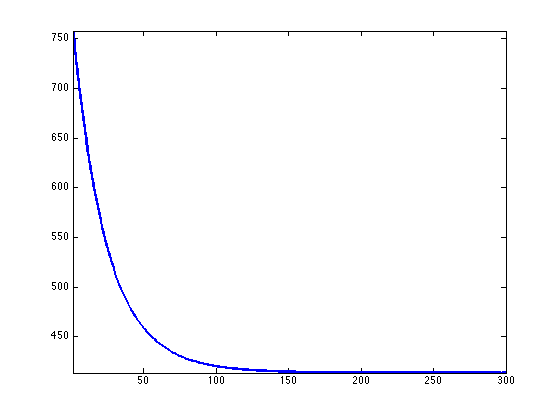Display the resulting denoised image.

clf;
imageplot(clamp(x));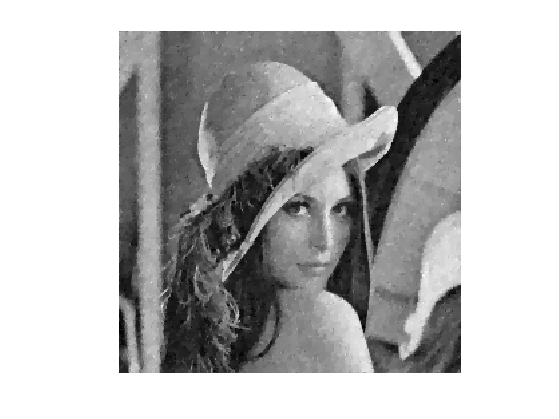## Constrained Optimization Using Projected Gradient Descent

We consider a linear imaging operator $$\Phi : x \mapsto \Phi(x)$$ that maps high resolution images to low dimensional observations. Here we consider a pixel masking operator, that is diagonal over the spacial domain.

To emphasis the effect of the TV functional, we use a simple geometric image.

n = 64;
name = 'square';


We consider here the inpainting problem. This simply corresponds to a masking operator. Here we remove the central part of the image.

a = 4;
Lambda = ones(n);
Lambda(end/2-a:end/2+a,:) = 0;


Masking operator $$\Phi$$. Note that it is symmetric, i.e. $$\Phi^*=\Phi$$

Phi  = @(x)x.*Lambda;
PhiS = @(x)Phi(x);


Noiseless observations $$y=\Phi x_0$$.

y = Phi(x0);


Display.

clf;
imageplot(x0, 'Original', 1,2,1);
imageplot(y, 'Damaged', 1,2,2);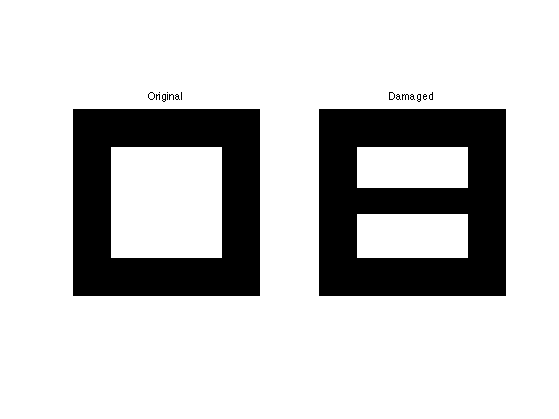We want to solve the noiseless inverse problem $$y=\Phi f$$ using a total variation regularization: $\umin{ y=\Phi x } J_\epsilon(x).$ We use the following projected gradient descent $x^{(k+1)} = \text{Proj}_{\Hh}( x^{(k)} - \tau_k \nabla J_{\epsilon}(x^{(k)}) )$ where $$\text{Proj}_{\Hh}$$ is the orthogonal projection on the set of linear constraint $$\Phi x = y$$, and is easy to compute for inpainting

ProjH = @(x,y) x + PhiS( y - Phi(x) );


Exercice 4: (check the solution) Display the evolution of the inpainting process.

exo4;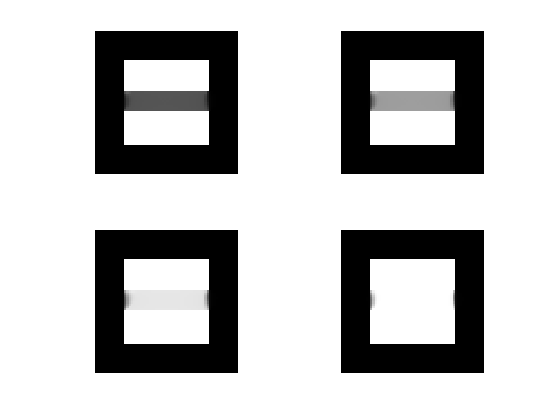Exercice 5: (check the solution) Try with several values of $$\epsilon$$.

exo5;